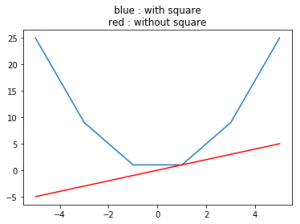# numpy.square() in Python

numpy.square(arr, out = None, ufunc ‘square’) : This mathematical function helps user to calculate square value of each element in the array.

Parameters :

```arr  : [array_like] Input array or object
whose elements, we need to square.
```

Return :

```An array with square value of each array.
```

Code #1 : Working

 `# Python program explaining ` `# square () function ` ` `  `import` `numpy as np ` ` `  `arr1 ``=` `[``1``, ``-``3``, ``15``, ``-``466``] ` `print` `(``"Square Value of arr1 : \n"``, np.square(arr1)) ` ` `  `arr2 ``=` `[``23` `,``-``56``] ` `print` `(``"\nSquare Value of arr2 : "``, np.square(arr2)) `

Output :

```Square Value of arr1 :
[     1      9    225 217156]

Square Value of arr2 :  [ 529 3136]
```

Code #2 : Working with complex numbers

 `# Python program explaining ` `# square () function ` ` `  `import` `numpy as np ` ` `  `a ``=` `4` `+` `3j` `print``(``"Square(4 + 3j) : "``, np.square(a)) ` ` `  `b ``=` `16` `+` `13j` `print``(``"\nSquare value(16 + 13j) : "``, np.square(b)) `

Output :

```Square(4 + 3j) :  (7+24j)

Square value(16 + 13j) :  (87+416j)```

Code #3 : Graphical Representation of numpy.square()

 `# Python program explaining ` `# square () function ` ` `  `import` `numpy as np ` `import` `matplotlib.pyplot as plt ` ` `  `a ``=` `np.linspace(start ``=` `-``5``, stop ``=` `5``, ` `                ``num ``=` `6``, endpoint ``=` `True``) ` `                 `  `print``(``"Graphical Representation : \n"``, np.square(a)) ` ` `  `plt.title(``"blue : with square\nred : without square"``) ` `plt.plot(a, np.square(a)) ` ` `  `plt.plot(a, a, color ``=` `'red'``) ` `plt.show() `

Output :

```Graphical Representation :
[ 25.   9.   1.   1.   9.  25.]
```References :
https://docs.scipy.org/doc/numpy-1.13.0/reference/generated/numpy.absolute.html
.

My Personal Notes arrow_drop_upIf you like GeeksforGeeks and would like to contribute, you can also write an article using contribute.geeksforgeeks.org or mail your article to contribute@geeksforgeeks.org. See your article appearing on the GeeksforGeeks main page and help other Geeks.

Please Improve this article if you find anything incorrect by clicking on the "Improve Article" button below.

Article Tags :

Be the First to upvote.

Please write to us at contribute@geeksforgeeks.org to report any issue with the above content.